# Mesh Denoising¶

Important: Please read the installation page for details about how to install the toolboxes. $\newcommand{\dotp}{\langle #1, #2 \rangle}$ $\newcommand{\enscond}{\lbrace #1, #2 \rbrace}$ $\newcommand{\pd}{ \frac{ \partial #1}{\partial #2} }$ $\newcommand{\umin}{\underset{#1}{\min}\;}$ $\newcommand{\umax}{\underset{#1}{\max}\;}$ $\newcommand{\umin}{\underset{#1}{\min}\;}$ $\newcommand{\uargmin}{\underset{#1}{argmin}\;}$ $\newcommand{\norm}{\|#1\|}$ $\newcommand{\abs}{\left|#1\right|}$ $\newcommand{\choice}{ \left\{ \begin{array}{l} #1 \end{array} \right. }$ $\newcommand{\pa}{\left(#1\right)}$ $\newcommand{\diag}{{diag}\left( #1 \right)}$ $\newcommand{\qandq}{\quad\text{and}\quad}$ $\newcommand{\qwhereq}{\quad\text{where}\quad}$ $\newcommand{\qifq}{ \quad \text{if} \quad }$ $\newcommand{\qarrq}{ \quad \Longrightarrow \quad }$ $\newcommand{\ZZ}{\mathbb{Z}}$ $\newcommand{\CC}{\mathbb{C}}$ $\newcommand{\RR}{\mathbb{R}}$ $\newcommand{\EE}{\mathbb{E}}$ $\newcommand{\Zz}{\mathcal{Z}}$ $\newcommand{\Ww}{\mathcal{W}}$ $\newcommand{\Vv}{\mathcal{V}}$ $\newcommand{\Nn}{\mathcal{N}}$ $\newcommand{\NN}{\mathcal{N}}$ $\newcommand{\Hh}{\mathcal{H}}$ $\newcommand{\Bb}{\mathcal{B}}$ $\newcommand{\Ee}{\mathcal{E}}$ $\newcommand{\Cc}{\mathcal{C}}$ $\newcommand{\Gg}{\mathcal{G}}$ $\newcommand{\Ss}{\mathcal{S}}$ $\newcommand{\Pp}{\mathcal{P}}$ $\newcommand{\Ff}{\mathcal{F}}$ $\newcommand{\Xx}{\mathcal{X}}$ $\newcommand{\Mm}{\mathcal{M}}$ $\newcommand{\Ii}{\mathcal{I}}$ $\newcommand{\Dd}{\mathcal{D}}$ $\newcommand{\Ll}{\mathcal{L}}$ $\newcommand{\Tt}{\mathcal{T}}$ $\newcommand{\si}{\sigma}$ $\newcommand{\al}{\alpha}$ $\newcommand{\la}{\lambda}$ $\newcommand{\ga}{\gamma}$ $\newcommand{\Ga}{\Gamma}$ $\newcommand{\La}{\Lambda}$ $\newcommand{\si}{\sigma}$ $\newcommand{\Si}{\Sigma}$ $\newcommand{\be}{\beta}$ $\newcommand{\de}{\delta}$ $\newcommand{\De}{\Delta}$ $\newcommand{\phi}{\varphi}$ $\newcommand{\th}{\theta}$ $\newcommand{\om}{\omega}$ $\newcommand{\Om}{\Omega}$

This tour explores denoising of 3-D meshes using linear filtering, heat diffusion and Sobolev regularization.

In :
using PyPlot
using NtToolBox
using Interpolations

WARNING: Method definition ndgrid(AbstractArray{T<:Any, 1}) in module NtToolBox at /Users/quentin/.julia/v0.5/NtToolBox/src/ndgrid.jl:3 overwritten at /Users/quentin/.julia/v0.5/NtToolBox/src/ndgrid.jl:3.
WARNING: Method definition ndgrid(AbstractArray{#T<:Any, 1}, AbstractArray{#T<:Any, 1}) in module NtToolBox at /Users/quentin/.julia/v0.5/NtToolBox/src/ndgrid.jl:6 overwritten at /Users/quentin/.julia/v0.5/NtToolBox/src/ndgrid.jl:6.
WARNING: Method definition ndgrid_fill(Any, Any, Any, Any) in module NtToolBox at /Users/quentin/.julia/v0.5/NtToolBox/src/ndgrid.jl:13 overwritten at /Users/quentin/.julia/v0.5/NtToolBox/src/ndgrid.jl:13.
WARNING: Method definition ndgrid(AbstractArray{#T<:Any, 1}...) in module NtToolBox at /Users/quentin/.julia/v0.5/NtToolBox/src/ndgrid.jl:19 overwritten at /Users/quentin/.julia/v0.5/NtToolBox/src/ndgrid.jl:19.
WARNING: Method definition meshgrid(AbstractArray{T<:Any, 1}) in module NtToolBox at /Users/quentin/.julia/v0.5/NtToolBox/src/ndgrid.jl:33 overwritten at /Users/quentin/.julia/v0.5/NtToolBox/src/ndgrid.jl:33.
WARNING: Method definition meshgrid(AbstractArray{#T<:Any, 1}, AbstractArray{#T<:Any, 1}) in module NtToolBox at /Users/quentin/.julia/v0.5/NtToolBox/src/ndgrid.jl:36 overwritten at /Users/quentin/.julia/v0.5/NtToolBox/src/ndgrid.jl:36.
WARNING: Method definition meshgrid(AbstractArray{#T<:Any, 1}, AbstractArray{#T<:Any, 1}, AbstractArray{#T<:Any, 1}) in module NtToolBox at /Users/quentin/.julia/v0.5/NtToolBox/src/ndgrid.jl:44 overwritten at /Users/quentin/.julia/v0.5/NtToolBox/src/ndgrid.jl:44.


## 3-D Triangulated Meshes¶

The topology of a triangulation is defined via a set of indexes $\Vv = \{1,\ldots,n\}$ that indexes the $n$ vertices, a set of edges $\Ee \subset \Vv \times \Vv$ and a set of $m$ faces $\Ff \subset \Vv \times \Vv \times \Vv$.

We load a mesh. The set of faces $\Ff$ is stored in a matrix $F \in \{1,\ldots,n\}^{3 \times m}$. The positions $x_i \in \RR^3$, for $i \in V$, of the $n$ vertices are stored in a matrix $X_0 = (x_{0,i})_{i=1}^n \in \RR^{3 \times n}$.

In :
X0,F = read_mesh("NtToolBox/src/data/elephant-50kv.off");


Number $n$ of vertices and number $m$ of faces.

In :
n = size(X0,2)
m = size(F,2);


Display the mesh in 3-D.

In :
figure(figsize=(10,10))
plot_mesh(X0, F);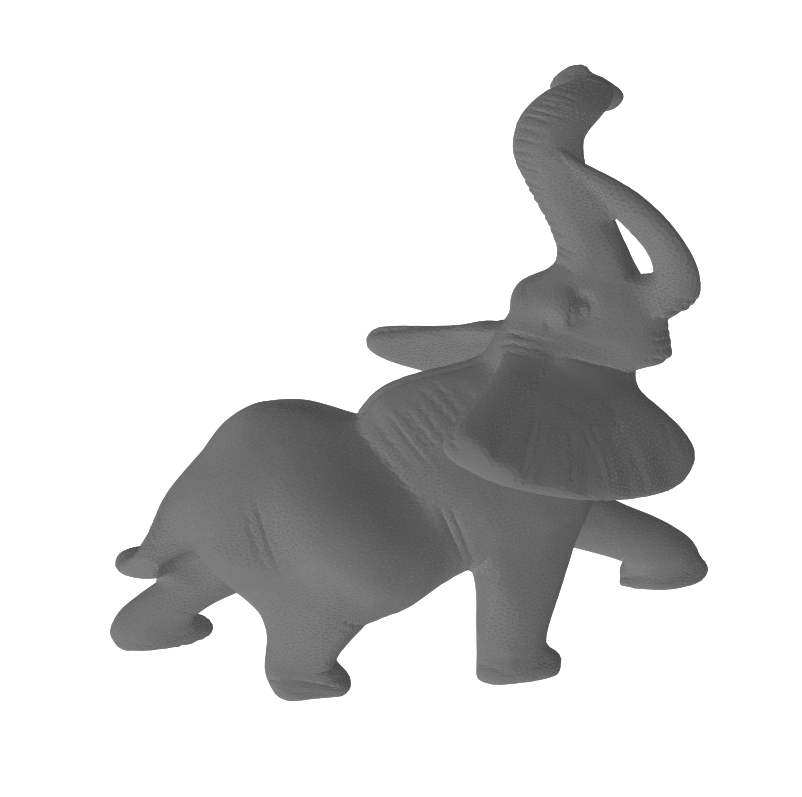## Noisy Mesh¶

We generate artificially a noisy mesh by random normal displacement along the normal. We only perform normal displacements because tangencial displacements do not impact the geometry of the mesh.

The parameter $\rho>0$ controls the amount of noise.

In :
rho = 0.015;


We compute the normals $N = (N_i)_{i=1}^n$ to the mesh. This is obtained by averaging the normal to the faces ajacent to each vertex.

In :
N = compute_normal(X0, F);


We create a noisy mesh by displacement of the vertices along the normal direction $$x_i = x_{0,i} + \rho \epsilon_i N_i \in \RR^3$$ where $\epsilon_i \sim \Nn(0,1)$ is a realization of a Gaussian random variable, and where $N_i \in \RR^3$ is the normal of the mesh for each vertex index $i$.

In :
noise = readdlm("noise")

Out:
24955×1 Array{Float64,2}:
-0.418846
-1.0755
-0.229355
-0.223954
0.915055
-0.99271
2.12146
-0.747118
0.953251
-1.24934
2.14039
0.808343
0.712551
⋮
1.65747
0.920563
-0.144339
-0.127088
0.328156
-0.27121
-1.09839
0.215768
-0.149779
0.427959
2.94325
0.54863 
In :
X = X0 + repeat(rho*randn(n)', outer=(3,1)).*N
X = X0 + repeat(rho*noise', outer=(3,1)).*N;


Display the noisy mesh.

In :
figure(figsize=(10,10))
plot_mesh(X, F);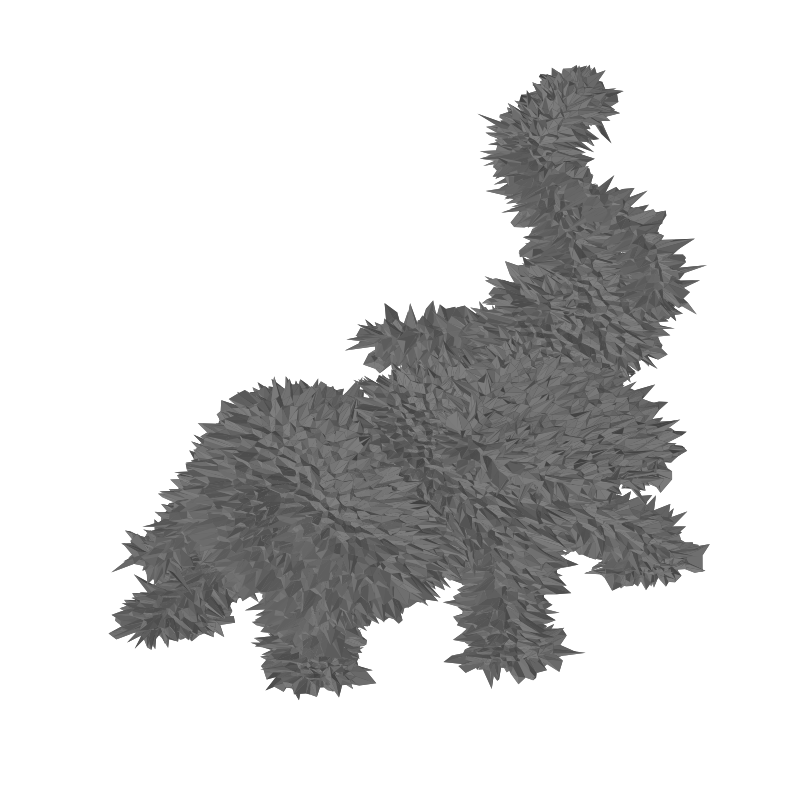We define linear operators that compute local averages and differences on the mesh.

First we compute the index of the edges that are in the mesh, by extracting pairs of index in the $F$ matrix.

In :
E = [F[[1, 2],:] F[[2, 3],:] F[[3, 1],:]];


Add the reversed edges. This defines the set of edges $\Ee$ that is stored in a matrix $E \in \{1,\ldots,n\}^{2 \times p}$.

In :
E = unique(sortcols([E E[2:-1:1,:]]), 2);


We keep only oriented pairs of index $(i,j)$ such that $i<j$, to avoid un-necessary computation.

In :
E0 = E[:,E[1,:] .< E[2,:]];


This defines a matrix $E \in \{1,\ldots,n\}^{2 \times p_0}$ where $p_0=p/2$.

In :
p0 = size(E0,2);


Display statistics of the mesh.

In :
print("#vertices = $n, #faces =$m, #edges = $p0")  #vertices = 24955, #faces = 49918, #edges = 74877 The weight matrix$W$is the adjacency matrix defined by $$W_{i,j} = \choice{ 1 \qifq (i,j) \in \Ee, \\ 0 \quad \text{otherwise.} }$$ Since most of the entries of$W$are zero, we store it as a sparse matrix. In : W = sparse(E[1,:],E[2,:],ones(size(E,2)));  Compute the connectivity weight vector$ d \in \NN^n $$$d_i = \sum_{j} W_{i,j}$$ i.e.$d_i$is the number of edges connected to$i$. In : d = vec(sum(W,1));  Display the statistics of mesh connectivity. In : plt[:hist](d, collect(minimum(d):maximum(d)+1));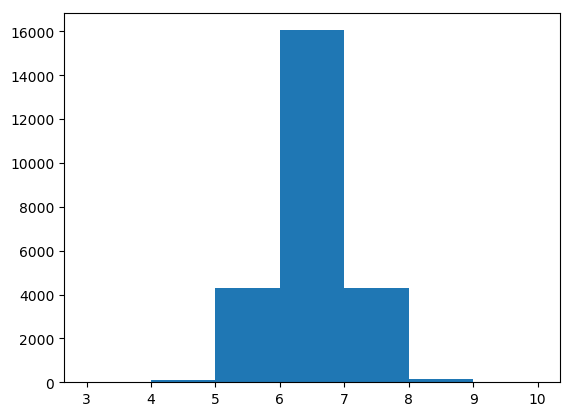Store in sparse diagonal matices$D$and$iD$respectively$D=\text{diag}_i(d_i)$and$D^{-1} = \text{diag}_i(1/d_i)$. In : D = sparse(collect(1:n), collect(1:n), vec(d)) iD = sparse(collect(1:n), collect(1:n), 1./vec(d));  The normalized weight matrix is defined as $$\tilde W_{i,j} = \frac{1}{d_i} W_{i,j},$$ and hence$\tilde W = D^{-1} W$. In : tW = iD*W;  It satisfies $$\forall i , \quad \sum_j \tilde W_{i,j} = 1,$$ i.e.$\tilde W \text{I} = \text{I}$where$\text{I} \in \RR^n$is the vector constant equal to one. The operator$\tilde W \in \RR^{n \times n} $, viewed as an operator$\tilde W : \RR^n \rightarrow \RR^n$, can be thought as a low pass filter. ## Laplacian and Gradient Operators¶ The un-normalized Laplacian is on the contrary a symmetric high pass operator $$L = D-W \in \RR^{n \times n}.$$ It satisfies$L \text{I} = 0$. In : L = D - W;  The gradient operator compute directional derivative along edges. It can be used to factor the Laplacian operator, but in practice it is never computed explicitely since it is never needed in numerical computation. To represent the gradient, we index the set of (oriented) edges$ \Ee_0 = (e_k)_{k=1}^{p_0} $where each edge is$e_k = (i,j) \in \{1,\ldots,n\}^2$with$i<j$. The gradient operator is a matrix$G \in \RR^{p_0 \times n}$defined as, for all$e_k=(i,j)$and all$\ell \notin \{i,j\}$, $$G_{k,i}=1, \quad G_{k,j}=-1, \quad G_{k,\ell}=0.$$ It is stored as a sparse matrix, and can be thought as a derivative operator$G : \RR^n \rightarrow \RR^{p_0} $that maps signal defined on vertices to differences located along directed edges. In : G = sparse([1:p0; 1:p0], [E0[1,:]; E0[2,:]], [ones(p0); -ones(p0)]);  Display the non-zero entries of$G$and$W$. In : figure(figsize=(15,7)) subplot(1, 2, 1) scatter(findn(W),findn(W), lw=.3) xlim(0,size(W,1)) ylim(1,size(W,2)) title("W") subplot(1, 2, 2) scatter(findn(G),findn(G),lw=.3) xlim(0,size(G,1)) ylim(1,size(G,2)) title("G");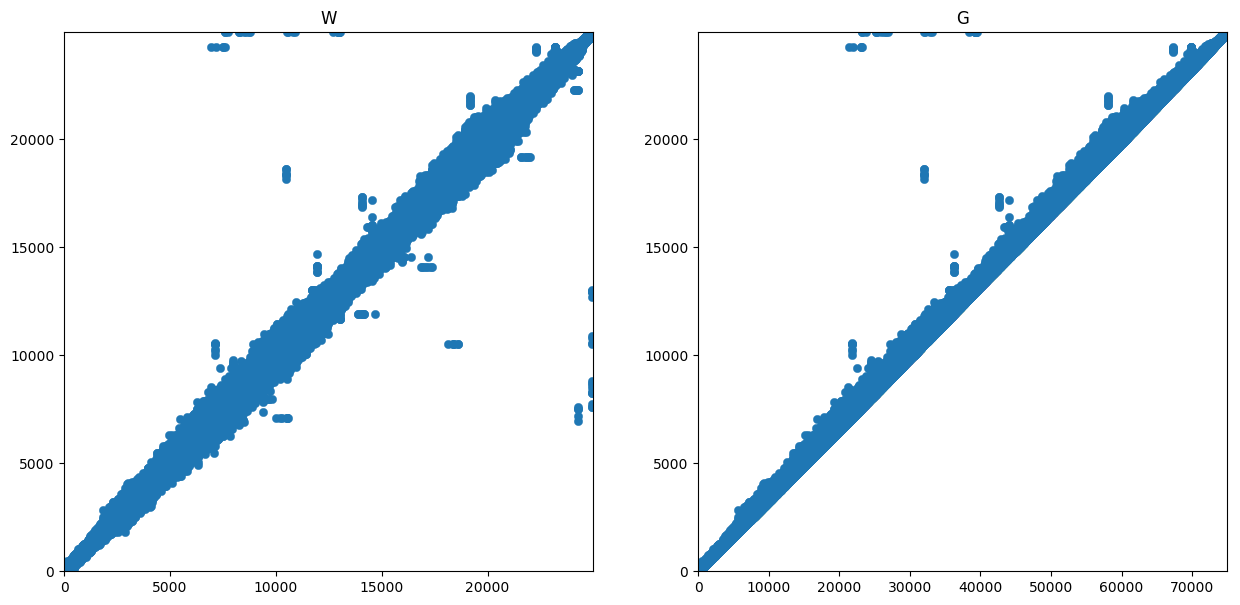The Laplacian can be factored as follow $$L = G^* G$$ where$G^*$is the transposed matrix (i.e. the adjoint operator, which can be thought as some kind of divergence). Check numerically that the factorization indeed hold. In : err = vecnorm(G'*G - L) print("Factorization error (should be 0) =$err")

Factorization error (should be 0) = 0.0

Note that this factorization shows that $L$ is a positive semi-definite operator, i.e. it satisfies

$$\dotp{L f}{f} = \norm{G f}^2 \geq 0.$$

If the mesh is connected, then only constant signals $f \in \RR^n$ satisfies $Lf=0$.

Note that this convention is the contrary to the usual convention of differential calculus, in which a Laplacian is a negative operator.

## Function Denoising with Filtering¶

A signal defined on the mesh is a vector $f \in \RR^n$, where $f_i \in \RR$ is the value at vertex $1 \leq i \leq n$.

Load a texture image $I$.

In :
M = load_image("NtToolBox/src/data/lena.png", 256);


Compute spherical coordinates $(\theta_i,\phi_i)$ for each vertex $x_{0,i}$ on the mesh.

In :
v = X0 - repeat(mean(X0,2), outer = (1,n))
theta = acos(v[1,:]./sqrt(sum(v.^2,1))')/pi
phi = (atan2(v[2,:],v[3,:])/pi + 1)/2.;


Interpolate the texture on the mesh.

In :
x = collect(linspace(0, 1, size(M,1)))
itp = interpolate((x,x), M', Gridded(Linear()))
f = zeros(length(theta))
for i in 1:length(theta)
f[i] = itp[theta[i], phi[i]]
end
f = rescale(f)
my_cmap = repeat(f, outer = (1,3));


Display the textured mesh.

In :
fig = figure(figsize = (10,10))
scatter3D(X0[1,:], X0[2,:], X0[3,:], c=my_cmap, s=30, lw=0)
axis("off")
gca()[:view_init](90,-90)
gca()[:dist] = 6;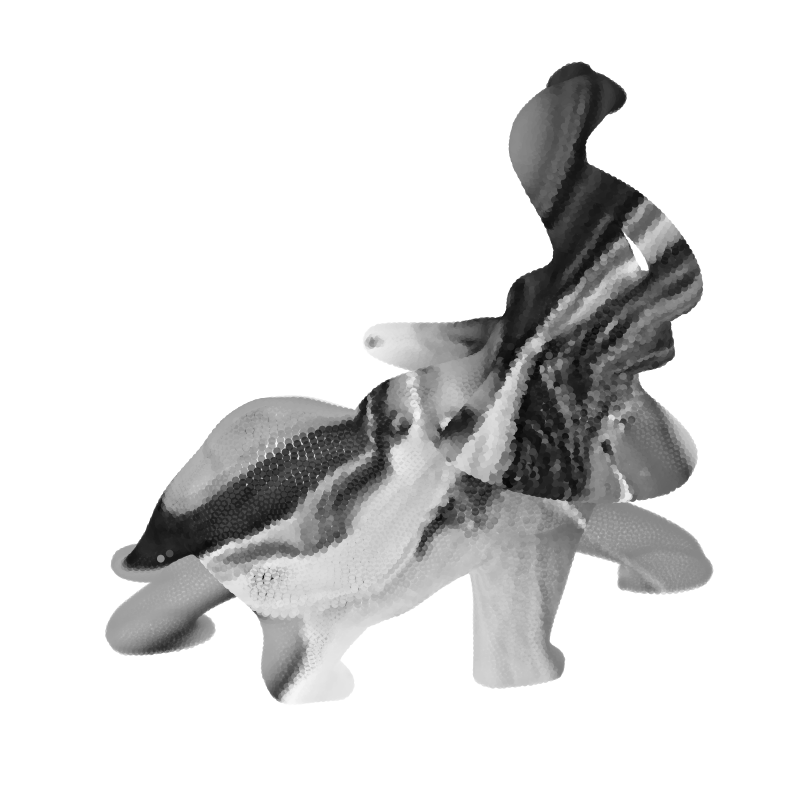The operator $\tilde W : \RR^n \rightarrow \RR^n$ can be used to smooth a function $f$, simply by computing $\tilde W f \in \RR^n$.

To further smooth the mesh, it is possible to iterate this process, by defining $f^{(0)} = f$ and

$$f^{(\ell+1)} = \tilde W f^{(\ell)}.$$

Note that one has $f^{(\ell)} = \tilde W^{\ell} f,$ but it is preferable to use the iterative algorithm to do the computations.

Exercise 1

Display the evolution of the image on the mesh as the number of iterations increases.

In :
include("NtSolutions/meshproc_3_denoising/exo1.jl")